Next: 2.1.2 Probabilistic PCA Up: 2.1 Principal component analysis Previous: 2.1 Principal component analysis

## 2.1.1 Neural networks for PCA

Principal components can be extracted using single-layer feed-forward neural networks (Sanger, 1989; Rubner and Tavan, 1989; Oja, 1989; Diamantaras and Kung, 1996). These networks learn unsupervised by using variants of the Hebbian rule. They provide an iterative solution to (2.5) and do not need the computation of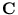, which could be computationally expensive (it is O(d 2n)). Networks extracting principal components further provide a biological basis for PCA. One example of such a PCA algorithm is Oja's rule.

Oja's algorithm (Oja, 1982) uses a single neuron with an input vector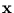, a weight vector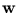, and an output y. The output can be written as y =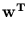(this corresponds to (2.1)). According to Oja's rule, after a training patternis presented, the weights change by a Hebbian term minus a forgetting function: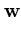(t + 1) =(t) +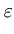y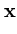-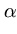y2(t) (2.6)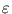is the Hebbian learning rate, and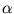is a constant. The forgetting term is necessary to bound the magnitude of. For the average update over all training patterns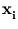, the fixed points of (2.6) can be computed. In turns out that they are the eigenvectors of the covariance matrix, and the eigenvector with the largest eigenvalue is the only stable point (Oja, 1982). Thus, Oja's rule extracts the principal component.Next: 2.1.2 Probabilistic PCA Up: 2.1 Principal component analysis Previous: 2.1 Principal component analysis
Heiko Hoffmann
2005-03-22